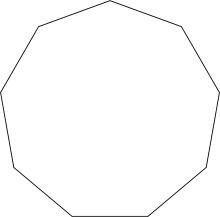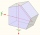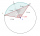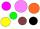# Nine-gon

Calculate the perimeter of a regular nonagon (9-gon) inscribed in a circle with a radius 13 cm.

x =  80.03 cm

### Step-by-step explanation:Did you find an error or inaccuracy? Feel free to write us. Thank you!Tips to related online calculators

#### You need to know the following knowledge to solve this word math problem:

We encourage you to watch this tutorial video on this math problem:

## Related math problems and questions:

• n-gonWhat is the side length of the regular 5-gon inscribed in a circle of radius 12 cm?
• Regular n-gonWhich regular polygon have a radius of circumscribed circle r = 10 cm and the radius of inscribed circle p = 9.962 cm?
• Hexagon ACalculate area of regular hexagon inscribed in circle with radius r=9 cm.
• n-gon IIWhat is the side length of the regular 5-gon circumscribed circle of radius 11 cm?
• PentagonCalculate the length of side, circumference and area of a regular pentagon, which is inscribed in a circle with radius r = 6 cm.
• Regular 5-gonCalculate the area of the regular pentagon with side 7 cm.
• NonagonCalculate the area and perimeter of a regular nonagon if its radius of the inscribed circle is r = 10cmCalculate the content of a regular 15-sides polygon inscribed in a circle with radius r = 4. Express the result to two decimal places.
• 30-gonAt a regular 30-gon the radius of the inscribed circle is 15cm. Find the "a" side size, circle radius "R", circumference, and content area.
• Inscribed and described circleFind the radii of a circle inscribed and circumscribed by a regular pentagon whose side measures 3 cm.
• Hexagon 5The distance of parallel sides of regular hexagonal is 61 cm. Calculate the length of the radius of the circle described to this hexagon.
• Hexagon in circleCalculate the radius of a circle whose length is 10 cm greater than the circumference of a regular hexagon inscribed in this circle.
• Length of the chordCalculate the length of the chord in a circle with a radius of 25 cm with a central angle of 26°.
• RT - inscribed circleIn a rectangular triangle has sides lengths> a = 30cm, b = 12.5cm. The right angle is at the vertex C. Calculate the radius of the inscribed circle.
• Hexagonal pyramidCalculate the surface area of a regular hexagonal pyramid with a base inscribed in a circle with a radius of 8 cm and a height of 20 cm.
• Circle and hexagonCalculate the radius of a circle whose circumference is 8.4 cm longer than the inscribed regular hexagon's circumference.
• Inscribed circleXYZ is right triangle with right angle at the vertex X that has inscribed circle with a radius 5 cm. Determine area of the triangle XYZ if XZ = 14 cm.